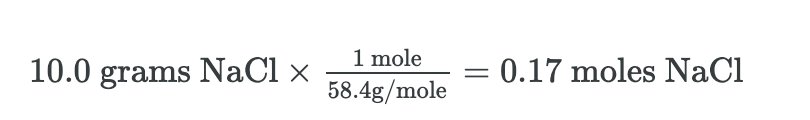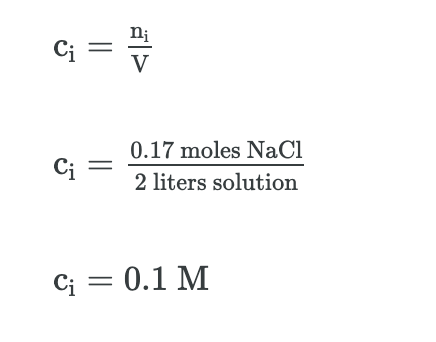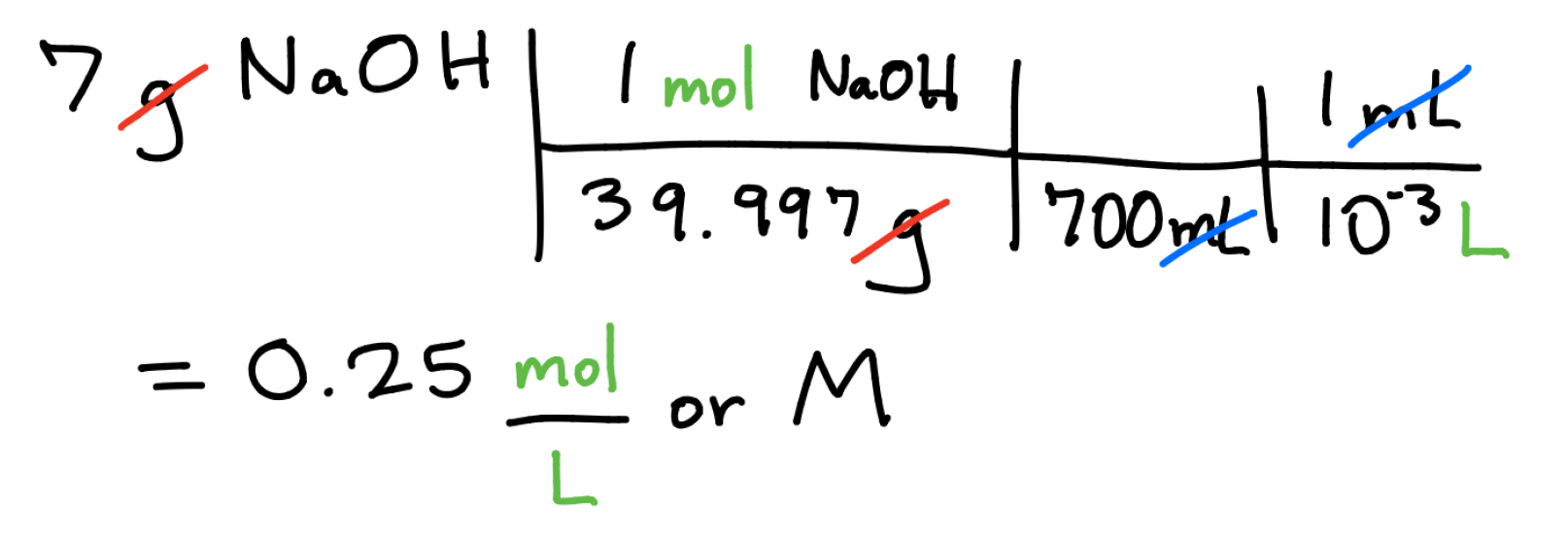Complimentary 1-hour tutoring consultation

MCAT Content / Solubility / Solubility Units Of Concentration

### Solubility Units of Concentration

Topic: Solubility

In chemistry, the concentration of a solution is often measured in molarity (M), which is the number of moles of solute per liter of solution.

Molar concentration (ci) is calculated by dividing the moles of solute (n) by the total volume (V) of the:

The SI unit for molar concentration is mol/m3. However, mol/L is a more common unit for molarity. A solution that contains 1 mole of solute per 1 liter of solution (1 mol/L) is called “one Molar” or 1 M. The unit mol/L can be converted to mol/m3 using the following equation:

1 mol/L = 1 mol/dm3 = 1 mol dm−3 = 1 M = 1000 mol/m3

To calculate the molarity of a solution, the number of moles of solute must be divided by the total liters of the solution produced. If the amount of solute is given in grams, we must first calculate the number of moles of solute using the solute’s molar mass, then calculate the molarity using the number of moles and total volume.

If there are 10.0 grams of NaCl (the solute) dissolved in water (the solvent) to produce 2.0 L of solution, what is the molarity of this solution?

First, we must convert the mass of NaCl in grams into moles. We do this by dividing by the molecular weight of NaCl (58.4 g/mole).Then, we divide the number of moles by the total solution volume to get concentration.The molarity is important to know when planning out experiments and reactions; the larger the molarity of a chemical, the higher the concentration. To determine the molarity of a substance, the number of moles of the object is divided by 1 liter (L) of the solvent. For example, if we wanted to know the concentration (M) of 7 grams of sodium hydroxide (NaOH) in 700 mL of water we could do the following calculation:When dilution occurs the volume of a solution is changed and this has an impact on the concentration of a solution. The equation c1V1 = c2V2, where c is concentration and V is volume can be used to calculate changes in concentration from volume changes.

Practice Questions

MCAT Official Prep (AAMC)

Chemistry Question Pack Passage 4 Question 20

Chemistry Question Pack Question 70

Section Bank C/P Section Passage 3 Question 22

Section Bank C/P Section Question 26

Section Bank C/P Section Passage 4 Question 34

Section Bank C/P Section Passage 9 Question 73

Sample Test C/P Section Question 15

Practice Exam 1 C/P Section Question 58

Practice Exam 2 C/P Section Passage 1 Question 3

Practice Exam 3 C/P Section Passage 5 Question 22

Practice Exam 4 C/P Section Passage 2 Question 7

Practice Exam 4 C/P Section Passage 5 Question 23

Key Points

• Molarity (M) indicates the number of moles of solute per liter of solution (moles/Liter) and is one of the most common units used to measure the concentration of a solution.

• Molarity can be used to calculate the volume of the solvent or the amount of solute.

• The relationship between two solutions with the same amount of moles of solute can be represented by the formula c1V1 = c2V2, where c is concentration and V is volume.

Key Terms

SI unit: the modern form of the metric system used extensively in the sciences (abbreviated SI from French: Système International d’Unités).

Molarity: the concentration of a substance in solution, expressed as the number moles of solute per liter of solution.

Concentration: the relative amount of solute in a solution

Solute: the solid dissolve in a liquid (solvent)

Solution: the product of dissolving a solute into a solvent

Billing Information